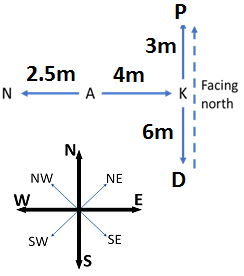# Direction Sense Test - Logical Reasoning (MCQ) Questions for Q. 29998

Q.  Study the information given below carefully, and answer the question that follow:
• On a stage, D, N, A and P are standing as described below facing North.
1) N is 2.5 m to the west of A.
2) K is 4 m to the right of A.
3) D is 6 m to the south of K.
4) P is 9 m to the north of D.

Who is to the south-west of P?

- Published on 15 May 17

a. A
b. D
c. K
d. Neither N nor A